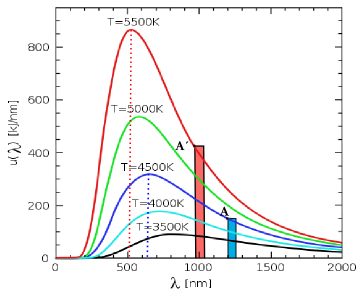# Wiens LawFigure 1. Blackbody radiation curves for a blackbody at four different temperatures, displaying the shift in peak wavelength for two different temperatures.

Wien's Law, sometimes called Wien's Displacement Law, is a law that determines at what wavelength the intensity of radiation emitted from a blackbody reaches its maximum point. After this point, the intensity decreases as temperature increases. This creates the characteristic shape of blackbody radiation curves. Wien's Law is expressed simply as:

Where is the wavelength in meters, and is the temperature in Kelvin. In this law temperature must be expressed on the absolute (Kelvin) scale. The displacement in Wien's law refers to the way that the position of the peak can be displaced with a change in temperature. Qualitatively this property can be seen quite simply. As an object is heated, it goes from red-hot to white-hot. This shows that as the temperature is increased, the wavelength that is emitted with the greatest intensity becomes smaller. The wavelength will decrease linearly as the temperature is increased. Thus, Wien's Law helps to illustrate the connection between colour and temperature. Note that Wien's Law only helps to determine at what wavelength the radiation of a blackbody peaks at. To determine the total energy emitted from the blackbody, the Stefan-Boltzmann law must be used.

Wien's law is useful in analyzing blackbody curves, but it is also used quite frequently to determine the temperature of hot radiant objects that cannot be easily measured, such as stars.